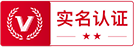## 土地估价师## 税务师# 2019年12月02日考研中医综合试题(第 1 套 - A型)

※考研中医综合在线模考>>开始

■ A型题

1. 阳损及阴的病机，主要指(  )
• A.阳气虚损，气化不利，水湿积聚
• B.外感温热阳邪，伤及阴液
• C.阳虚不能制阴，阴寒内盛
• D.阳气虚损，阴液化生不足

2. “主液”的腑是()
• A.胃
• B.胆
• C.小肠
• D.大肠

3. 扶正与祛邪兼用适用于(  )
• A.正气虚，邪气也不盛
• B.邪气盛，正气末衰
• C.邪气盛，正气也虚
• D.邪盛正虚，但正气尚耐攻伐

4. “泌别清浊”，属于()
• A.胃的生理功能
• B.大肠的生理功能
• C.小肠的生理功能
• D.肾的生理功能

5. 气大量丢失的病理变化为(  )
• A.气虚
• B.气滞
• C.气闭
• D.气脱

6. 临证所见“面黑齿长而垢，腹胀闭，上下不通”，其病机是(  )
• A.太阳之脉终结
• B.少阳之脉终结
• C.阳明之脉终结
• D.少阴之脉终结

7. 手厥阴经分布在(  )
• A.上肢外侧前缘
• B.上肢内侧前缘
• C.上肢内侧中线
• D.上肢外侧中线

8. 过度恐惧对气机的影响是(  )
• A.气结
• B.气消
• C.气上
• D.气下

9. 元气不足，脏腑功能减退，卫外不固形成的病理变化是(  )
• A.气虚
• B.气滞
• C.气闭
• D.气逆

10. 气滞血瘀多与哪一脏的功能失调有关(    )
• A.肝
• B.心
• C.肺
• D.脾

11. 七情內伤致病，首先损伤(  )
• A.肝
• B.心
• C.肺
• D.脾

12. 区分五脏、六腑、奇恒之府的最主要依据是(  )
• A.解剖形态
• B.所在部位
• C.阴阳属性
• D.功能特点

13. 五行相乘，下列哪种说法是正确的(  )
• A.母气有余而乘其子
• B.子气有余而乘其母
• C.气有余则制己所胜
• D.气有余则乘己所不胜

14. “痛痹”是以什么邪气为主引起的(  )
• A.风邪
• B.寒邪
• C.湿邪
• D.暑邪

15. 根据阴阳学说，药用五味中属阳的是(    )
• A.辛、甘、酸
• B.酸、苦，咸
• C.辛、苦、甘
• D.辛、甘、淡

16. 寒邪客肺证与饮停于肺证的区别有(  )
• A.有无咳嗽
• B.有无气喘
• C.痰质稀
• D.有无既往发作史

17. 在下列症状中，哪项不符合脾肺气虚的临床表现(  )
• A.咳喘短气，痰稀色白
• B.胸闷，善太息
• C.食欲不振，腹胀便溏
• D.倦怠乏力，少气懒言

18. 肝血虚证不见(  )
• A.关节拘急
• B.肢体麻木
• C.手足颤动
• D.颈项强直

19. 我国最早的脉学专书《脉经》提出多少种脉象(  )
• A.二十八种
• B.二十七种
• C.二十四种
• D.十六种

20. 大便夹有不消化食物，酸腐臭秽者，多因(    )
• A.大肠湿热
• B.寒湿内盛
• C.伤食积滞
• D.脾胃虚弱

21. 失神与神气不足的共同表现是(  )
• A.言语失伦
• B.表情淡漠
• C.倦怠乏力
• D.面色晦暗

22. 短缩舌与痿软舌的共同病机是(  )
• A.寒凝筋脉
• B.痰浊内阻
• C.风痰阻络
• D.气血俱虚

23. 脾病的常见症状，除外哪项均是(  )
• A.腹胀腹痛
• B.恶心嗳气
• C.浮肿
• D.泄泻

24. 外感秽浊之气，热毒内盛可见(  )
• A.白腻苔
• B.黄腻苔
• C.积粉苔
• D.灰黑干燥苔

25. 身热不扬的表现，多由于(  )
• A.脾气虚损
• B.阴经郁热
• C.外感风热
• D.湿遏热伏

26. 《素问．刺热篇》把哪脏与额加以联系(    )
• A.肝
• B.肺
• C.心
• D.脾

27. 下列哪项不属于中焦病证的临床表现(    )
• A.面目俱赤
• B.舌謇肢厥
• C.口干咽燥
• D.胸闷不饥

28. 语言错乱，说后自知，为(  )
• A.错语
• B.谵语
• C.夺气
• D.独语

29. 补气养阴，清火生津宜首选的药物是(    )
• A.山药
• B.西洋参
• C.太子参
• D.沙参

30. 不可割取地上部分入药的是(  )
• A.稀莶草
• B.灯心草
• C.泽兰
• D.龙胆草

31. 柿蒂的性味是(  )
• A.苦平
• B.苦寒
• C.辛热
• D.辛凉

32. 具有清肝养血、明目退翳功效的药物是(    )
• A.谷精草
• B.密蒙花
• C.菟丝子
• D.青葙子

33. 功能收敛止血，固精止带，制酸止痛，收湿敛疮的药物是(  )
• A.牡蛎
• B.瓦楞子
• C.乌贼骨
• D.赤石脂

34. 入汤剂宜包煎的药物是(  )
• A.自然铜
• B.苏木
• C.虎杖
• D.五灵脂

35. 蟾酥內服的用量是(  )
• A.0．015~0．03g
• B.0．3一0．6g
• C.0．002~0．004g
• D.0．1一0．2g

36. 代赭石除具有平肝潜阳作用外，还能(    )
• A.收敛固涩
• B.镇惊安神
• C.清肝明目
• D.降逆止呕

37. 不是源于蔷薇科植物的药是(  )
• A.地榆
• B.山楂
• C.玫瑰花
• D.荔枝核

38. 生地黄的性味是(  )
• A.辛，苦、寒
• B.苦，淡、寒
• C.酸，苦、寒
• D.甘，苦、寒

39. 肝郁血虚，脾失健运，见两胁作痛，神疲食少，脉虚弦者，治宜选用(  )
• A.一贯煎
• B.越鞠丸
• C.逍遥散
• D.四逆散

40. 体倦气短，口渴多汗，舌燥咽干，脉虚细者，治宜选用(  )
• A.生脉散
• B.清暑益气汤
• C.当归补血汤
• D.竹叶石膏汤

41. 半夏厚朴汤的功用是(  )
• A.行气降逆，燥湿化痰
• B.疏肝散结，燥湿化痰
• C.行气疏肝，温胃散寒
• D.行气散结，降逆化痰

42. 六味地黄丸不适宜的主治证为(  )
• A.耳鸣耳聋，头目眩晕
• B.腰膝酸软，盗汗遗精
• C.脑中热痛，头面如醉
• D.骨蒸潮热，手足心热

43. 再造散的组成中含有的药物是(  )
• A.熟地黄、怀牛膝
• B.生附子、炮干姜
• C.熟附子、煨生姜
• D.怀牛膝、枸杞子

44. 清气化痰丸制备申加入的是(  )
• A.水
• B.蜜
• C.梨汁
• D.姜汁

45. 镇肝熄风汤中少佐茵陈，意在(  )
• A.清泄肝热
• B.疏解肝郁
• C.疏肝泄热
• D.清热利湿

46. 芍药汤与白头翁汤共有的药物是(  )
• A.黄连
• B.黄芩
• C.黄连、黄柏
• D.黄连、黄芩

47. 旋覆代赭汤中所用姜是(  )
• A.生姜
• B.干姜
• C.炮姜
• D.煨姜

48. 哪项不是八正散主治证的症状(  )
• A.小便涩痛
• B.小腹急满
• C.恶心呕吐
• D.舌苔黄膩

49. 当归六黄汤的功用是(  )
• A.泻火解毒，滋阴养血
• B.滋阴泻火，养血和血
• C.养血除烦，泻火坚阴
• D.滋阴泻火，固表止汗

50. 败毒散中配伍少量人参，意在(  )
• A.益气补虚
• B.扶正祛邪
• C.益气固表
• D.使祛邪不伤正

51. 阳和汤除有熟地黄、麻黄、炮姜炭、鹿角胶、生甘草外还有(  )
• A.桂枝、半夏
• B.肉桂、白芥子
• C.肉桂、附子
• D.桂枝、紫苏子

52. 复元活血汤的君药为(  )
• A.柴胡、当归
• B.柴胡、大黄
• C.红花、桃仁
• D.当归、红花

53. 一老叟于沐浴时，突发少腹拘急冷痛，喜暖喜按，得温痛减，舌苔白，脉沉紧，治宜选用(  )
• A.乌头桂枝汤
• B.附子理中丸
• C.附子粳米汤
• D.暖肝煎

54. 患者每遇情志刺激诱发，突然呼吸短促，但喉申痰声不著，气憋，胸闷胸痛，咽中如窒，心悸，苔薄，脉弦。其治法是(  )
• A.理气化痰平喘
• B.清热泻火理气
• C.疏肝清肺化痰
• D.开郁降气平喘

55. 与眩晕发生关系密切的脏腑是(  )
• A.肺、脾、肾
• B.心、肝、肾
• C.肝、肾、脾
• D.肺、胃、肾

56. 患者哮证日久，反复发作，发时喉中痰鸣如鼾，声低，气短不足以息，咳痰清稀，面色苍白，汗出肢冷，舌淡白，脉沉细者，治宜选用(  )
• A.苏子降气汤
• B.金匱肾气丸
• C.射干麻黄汤
• D.七味都气丸

57. 长期抑郁，现眩晕心悸，少寐，心烦易怒，舌红，苔少，脉弦细数，治疗方剂宜选(  )
• A.右归丸
• B.左归丸
• C.六味地黄丸
• D.滋水清肝饮

58. 患者病痰饮，心下坚满而痛，自利，利后反快，虽利心下续坚满，口舌干燥，舌苔黄腻，脉沉弦。治疗宜首选(  )
• A.甘遂半夏汤
• B.己椒苈黄丸
• C.小陷胸汤
• D.小半夏加茯苓汤

59. 肾虚腰痛，日久不愈，无明显阴阳偏虚，宜选用(  )
• A.左归丸
• B.右归丸
• C.大补阴丸
• D.青娥丸

60. 下列哪项不是鼓胀晚期的常见并发症(    )
• A.吐血
• B.黄疸
• C.水肿
• D.中风

61. 下列哪一项不是肺癆的主症(  )
• A.咳血
• B.咳嗽
• C.盗汗
• D.胸闷胁胀

62. 患者，女，36岁，潮热盗汗，虚烦少寐，五心烦热，口渴，月经不调，舌红少苔，脉细数。治疗应当(  )
• A.清里泻热
• B.养心安神敛汗
• C.滋阴降火
• D.滋补肝肾

63. 胸闷隐痛，时作时休，心悸气短，倦怠懒言，面色少华，头晕目眩，遇劳加重，舌淡胖有齿印，脉结代者，治疗选用(  )
• A.生脉散
• B.天王补心丹
• C.炙甘草汤
• D.人参养荣汤

64. 患者眩晕耳鸣，头痛且胀，面色潮红，急躁易怒，少寐多梦，目赤口苦，便干溲赤，舌苔黄燥，脉象弦数，其治疗主方应为(  )
• A.天麻钩藤饮
• B.镇肝熄风汤
• C.龙胆泻肝汤
• D.当归芦荟丸

65. 用槟榔南瓜煎治疗绦虫病，其服药顺序是(  )
• A.槟榔、南瓜子、玄明粉
• B.槟榔、玄明粉、南瓜于
• C.南瓜子、玄明粉、槟榔
• D.南瓜子、槟榔、玄明粉

66. 用麻子仁丸治疗的是(  )
• A.气秘
• B.热秘
• C.气虚秘
• D.冷秘

67. 哮证发作期的主要病因病机是(  )
• A.外邪侵袭，触动伏痰
• B.宿痰内伏于肺
• C.邪客于肺，气道不利
• D.痰瘀相搏，气道被阻

68. 噎膈治疗要加入滋阴润燥之品的病期是(  )
• A.初期
• B.中期
• C.恢复期
• D.后期

69. 水肿的发病病机申，其标在肺，其制在脾，其本在(  )
• A.膀胱
• B.三焦
• C.肾
• D.心

70. 痢疾的病位在(  )
• A.胃
• B.肠
• C.肾
• D.脾

71. 患者平素头晕头痛，耳呜目眩，少寐多梦，突然发生口舌喁斜，舌强语謇，半身不遂，舌红，脉弦细数。治疗宜用(  )
• A.苏合香丸
• B.镇肝熄风汤
• C.安宫牛黄丸
• D.补阳还五汤

72. 下列哪项不是时行感冒的特点(  )
• A.起病急
• B.全身症状重
• C.易化热人里，变生他病
• D.常见痰热郁肺症状

73. 犊鼻穴下3寸，胫骨前嵴外开一横指是(    )
• A.下巨虚
• B.上巨虚
• C.光明
• D.足三里

74. 患者，男，22岁。发热恶寒，寒重热轻，头痛身痛，鼻塞流涕，咳嗽，咳痰清稀，舌苔薄白，脉浮紧。治疗应首选(  )
• A.手太阴、手阳明经穴
• B.手少阴、手太阳经穴
• C.手太阴、手少阳经穴
• D.手太阴、足太阳经穴

75. 患者，男，30岁。口角歪向右侧，左眼不能闭合2天，左侧额纹消失，治疗应取经穴为(  )
• A.手、足少阳经和手、足太阴经
• B.手、足太阴经和手、足太阳经
• C.手、足厥阴经和手、足阳明经
• D.手、足阳明经和手、足太阳经

76. 肘横纹中，肱二头肌腱尺侧缘的穴位是(    )
• A.曲池
• B.曲泽
• C.少海
• D.尺泽

77. 患者，女，45岁。失眠2年，经常多梦少寐，入睡迟，易惊醒，平常遇事惊怕，多疑善感，气短头晕，舌淡，脉弦细。治疗除取主穴外，还应加(  )
• A.心俞、肾俞、太溪、足三里
• B.心俞、厥阴俞、脾俞
• C.心俞、胆俞、大陵、丘墟
• D.肝俞、间使、太冲

78. 患者，男，55岁，患者3天前自觉右胁背部疼痛，并逐渐出现疱疹，呈现带状分布，疼痛较剧，口干苦、大便干、小便黄，脉弦，舌红苔黄。根据病情应取哪组经脉或腧穴为主(  )
• A.手足阳明经
• B.局部阿是穴及相应夹脊穴
• C.手足太阴经
• D.手足太阳经

79. 有关针刺治疗落枕的说法不正确的是(    )
• A.毫针刺法
• B.可加灸法
• C.远端穴位可持续捻转
• D.捻针时嘱患者不可活动颈项部

80. 患者，男，67岁，患者突发右侧肢体活动不利，言语不清，口角流涎，伸舌右偏、口角喁斜，血压180／100mmHg，大便3日未行，脉弦，舌暗红苔黄腻。此病例根据症、舌、脉应辨证为(  )
• A.风阳上扰
• B.气虚血瘀
• C.肝胆火旺
• D.痰热腑实

※考研中医综合在线模考>>查看答案
 留言与评论（共有 条评论）

 验证码：#### 标签列表

http://www.zuoti.com/

### var _hmt = _hmt || []; (function() { var hm = document.createElement("script"); hm.src = "https://hm.baidu.com/hm.js?db7dc45ce37deaf748e02c0394b4349b"; var s = document.getElementsByTagName("script"); s.parentNode.insertBefore(hm, s); })(); | 闽ICP备20005157号-1